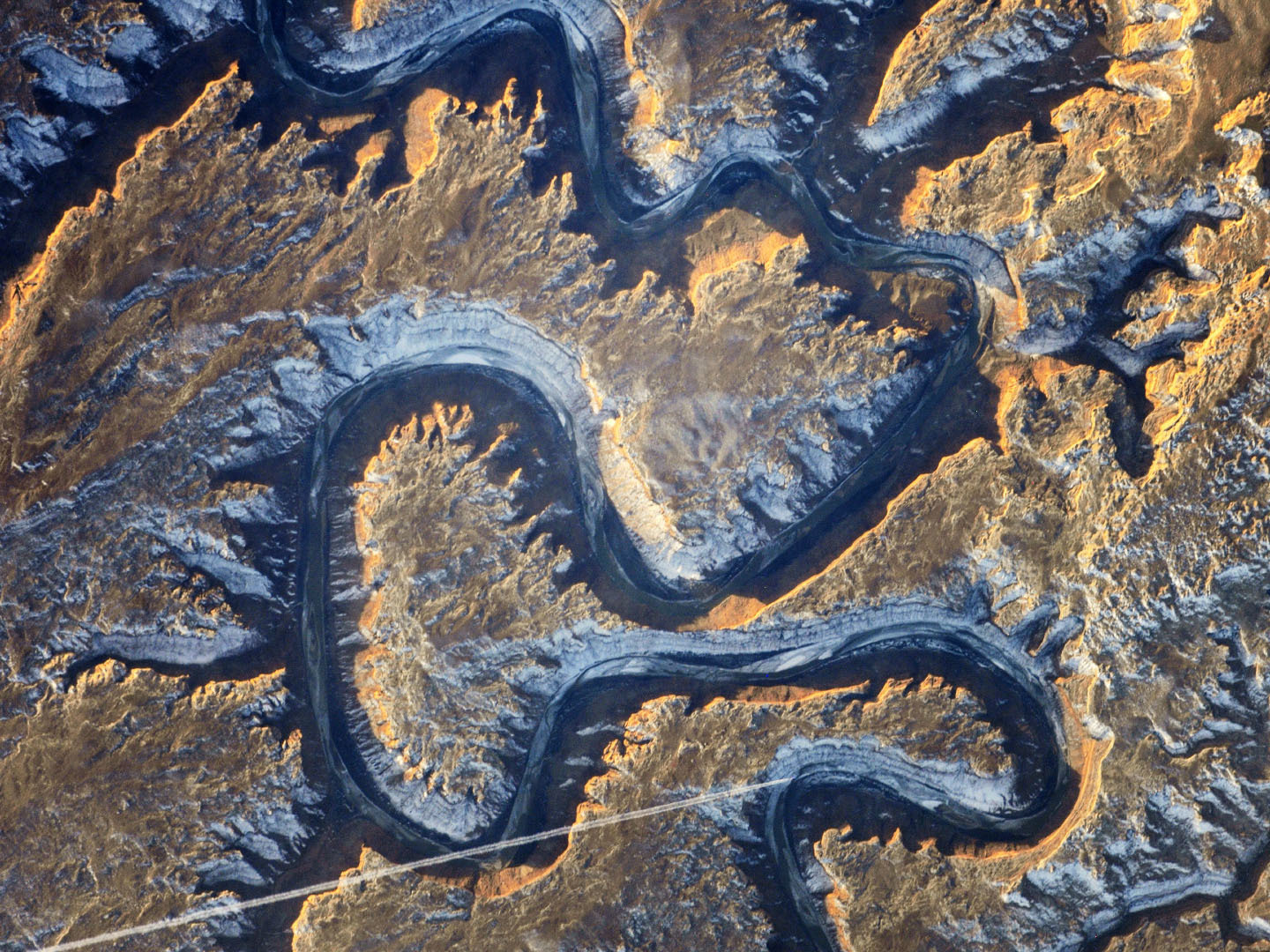Snider
CFD using Power

1. # ?

How is this method different than the Vortex Method?
The vortex method transforms the Cartesian/linear motion coordinates to Polar or Rotational Coordinate system. Conservation of mass, momentum and energy are carried out in this coordinate system. Conservation of Power CFD allows the use of both coordinate systems simultaneously.
2. # ?

Resistance varies with velocity linearly, energy varies with the velocity squared, will energy be correct at each point in the model?
Power varies with the cube of velocity: Power = Q^2R. Thus if Q is correct one could back calculate velocity and assure that energy is also conserved. Also, Conservation of Energy states: "In a closed system the total energy is a constant and this energy can transform from one form to another." To comply with conservation of energy, the time rate of transformation (power) of the "source" or decreasing energy form(s) must equal the time rate of transformation of the "sink" or increasing energy form(s) to maintain a constant total amount of energy in the closed system over time. Or the time rate of change (in the closed system) of the total energy is zero, since the total energy is a constant. The derivitive of a constant is zero. I call this "Conservation of Power" rather than saying all the above. It could be called: "The First Time Derivative of the rate of change of energy in the "Conservation of Energy"" equation. The key is to conserve the total energy in each element (a closed system) and within the model. Interestingly both power and energy are an extension of F = ma. It could be called: " Snider's Law." Just kidding. But by definition, Conservation of Power implies Conservation of Energy in a closed system. Electrical engineers call this "Circuit Analysis." See the FAQ download for more....
3. # ?

Why conserve power? Nobody conserves power in CFD.
Conservation of energy states that in a closed system there is a constant amount of total energy and energy can transform from one form to another within the closed system. Pressure (potential) transforming to velocity (Kinetic Energy) is an example. The time rate of change from one form of energy to another is power. Thus to assure conservation of energy (the total energy is a constant) the time rate of change in a source energy form must equal the time rate of change of the sink energy form. Fluid Power designers use Poiseuille's Equation and or empirical formulas based on velocity, change in height, etc for each of the components in the system. They then add them together to model the change in pressure as a flow moves through the system. Thus they can size the prime fluid movers as well as other system components. This process is a crude form of CFD since designers use software programs to aid their calculations. Power CFD builds on this to find the elemental forces, stresses and motions of a flow field that cause the pressure or flow rate change. See the physics section for the development of this equation for "resistance" in non Tensor form.
4. # ?

Does this modeling method’s work flow differ from what I do now?
No. Geometry, Boundary Conditions, Knowns and Unknowns remain the same as Navier-Stokes based models.
1. # ?

Fluid flow is described by elliptic, parabolic and hyperbolic partial differential equations depending on flow conditions--How will the results match real flows?
Elliptic equations are well suited to describe equilibrium states, Parabolic equations describe processes that behave essentially like heat diffusing through a solid and the solutions of hyperbolic equations are "wave-like." A two or three dimensional array of foregoing equations have the characteristic that a disturbance at one point in will have an effect or propagate to the other points. So too will a resistor array, since the resistors are "joined" by the stress state or tensor values around each resistor element. Pressure and Velocity gradients will be "passed" through the resistor array just as in a Navier-Stokes based method using partial differential equations or in a real flow field.
2. # ?

Yes, the calculation of resistance is simpler than solving PDE models, but the method uses a lot of computational resources to reformulate the resistors. Will it be better than current methods?
I do not know. I do not have working code. It should: Since flow conditions determine "mesh" or resistor size and position, the method will eliminate mesh sensitivity and convergence issues. Compressible flow models will be more robust and hopefully accurate. The method also will eliminate the operator experience factor which is very costly to develop currently. It should better model high speed jets in compressible flow domains, “bubble” behavior in flow over wings, etc. Since the “meshing” is dynamic, moving boundaries and geometries can be easily modeled—Aero acoustic problems, Avian flight, fish swimming, blood flow in an elastic vein, air movement in alveoli of the lungs, reciprocating engines and the list goes on and on. Current methods have a hard time with turbulence’s wide scale temporal and spatial range. A “library” of resistor elements would simplify the “digitization” of this phenomena addressing the problem of sub-element scale modeling.